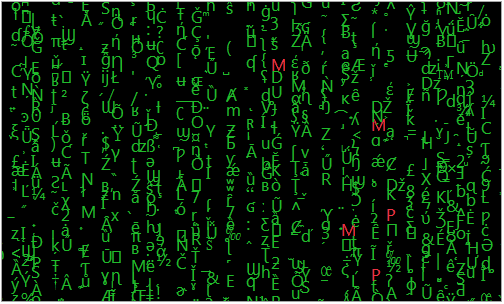Our World Statistics Day conversations have been a great reminder of how much statistics can inform our lives. Do you have an example of how statistics has made a difference in your life? Share your story with the Community!
Choose Language Hide Translation Bar
What is the JSL Matrix?Animated gif of random green letters with random red J, M, and P characters

JMP has a powerfull set of matrix primitives in the JSL scripting language. Here's an introduction to creating and using 1D and 2D matrices in JSL.

A JSL matrix constant for a 3 row by 2 column matrix looks like this; notice the spaces between elements on a row and the commas between rows.

``````constant = [
1 2,
3 4,
5 6
];``````

When you run that code it will display like this in the log window (and you can write it on one line too):

`[1 2, 3 4, 5 6]`

The square brackets define a constant that is created when the JSL is parsed. You can't use other JSL variables between the square brackets because the parser runs before the variables exist (more on that in a moment). Two more examples using 1D matrices:

``````RowVector = [1 2];
ColVector = [3,4];``````

JSL has several functions for creating matrices. I usually use J( nRows, nCols, init ), with 0 for the 3rd init value.

```squareOnes = J(5);
[1 1 1 1 1,
1 1 1 1 1,
1 1 1 1 1,
1 1 1 1 1,
1 1 1 1 1]

diagOnes = identity(5);
[1 0 0 0 0,
0 1 0 0 0,
0 0 1 0 0,
0 0 0 1 0,
0 0 0 0 1]

colsAndRows = J(3,2,random integer(1,6));
[2 6, 2 5, 5 2]```

You can also build matrices by concatenating values together, horizontally or vertically. The same concatenation operators will concatenate matrices that have compatible sizes (not shown here).

```a=1;b=2;c=3;d=4;e=5; // now we can use variables as follows

row1 = a::e; // row
[1 2 3 4 5]

col1 = (a::e)`; // column - transpose operator
[1, 2, 3, 4, 5]

row2 = a||22||c||44||e; // row - concatenate horizontal
[1 22 3 44 5]

col2 = a|/222|/c|/444|/e; // column - concatenate vertical
[1, 222, 3, 444, 5]```

Than tiny little ` character is at the top left of a US keyboard and is the matrix transpose operator. The :: operator makes a matrix of integers. You can multiply by a scalar and add a scalar to get the result matrix you need.

```(0::9)*.1+100
[100 100.1 100.2 100.3 100.4 100.5 100.6 100.7 100.8 100.9]```

Matrices are subscripted in the ususal way. Matrix subscripts start at 1 in JSL, not 0 as in some languages.

```M = [
11 12 13 14 15,
21 22 23 24 25,
31 32 33 34 45
];

write(M);
[	11 12 13 14 15,
21 22 23 24 25,
31 32 33 34 45]

write(M[2,3])
23```

And they can be sliced and diced in powerful ways:

```write(M[2::3, 1::2]); // return a 2x2 sub-matrix
[21 22, 31 32]

write(M[1,0]); // 0 means the entire dimension
[11 12 13 14 15]

write(M[0,2::3]); // various combinations
[12 13, 22 23, 32 33]```

There is a matrix function in JSL called shape that is sometimes useful for reshaping a row or column vector into a 2D matrix. There are two multiply operators for matrices, one for matrix algebra multiplies ( m1 * m2 ) and another for element-by-element multiplication:

```[1 2, 3 4] :* [2 3, 4 5]
[2 6, 12 20]```

Remember that :* operator if you get really unexpected results multiplying two images together!

You can assign values to matrix elements or slices too:

```mat = J(2,3,.); // 2 rows, three columns, all missing
mat[1,1] = 7; // the top left corner
mat[0,2::3] = [5 9, 13 15]; // all rows, columns 2 and 3
write(mat); // notice the missing value that never got set
[7 5 9, . 13 15]```

Finally, here's the code for the animated gif that has almost nothing to do with the topic. It uses matrices in surprising ways to manipulate images and characters and probably isn't the example you want to start with.

``````matgif = "\$desktop/mat.gif";
gb = Graph Box(framesize(500,300));
gb << setBackgroundColor( "black" );
gb << setGraphicsScript(
Text Color( "green" );
For( x = -10, x <= 110, x += Random Integer( 2, 4 ),
For( y = -10, y <= 110, y += Random Integer( 3, 7 ),
c = random integer(31,1000);
textcolor("green");
if( random integer(1,10000)<60,
textcolor("red");
c = blobtomatrix(chartoblob(substr("JMP", random integer(1,3),1)),"uint",1,"big")
);
Text( {x, y}, blobtochar(matrixtoblob(c::c,"uint",2,"big"),encoding="utf-16be") )
);
);
);
New Window( "x", gb );
duration=80;//ms
animation = gb[framebox(1)]<<getpicture;
animation<<setFrameDuration(duration);//ms
for( ipic=1,ipic<=7,ipic++,
gb << inval; gb<<updatewindow;
p = gb[framebox(1)]<<getpicture;
animation<<setFrameDuration(duration);//ms
animation<<setPixels(p<<getpixels);
);
animation<<saveImage(matgif,"gif");
open(matgif);
filesize(matgif);``````

Article Labels

There are no labels assigned to this post.

Article Tags
Level VI

thanks for the instruction.  The code on how to create an animated GIF was really helpful, too!  I had been trying to figure that out recently.Staff (Retired)

welcome, and thanks!Staff (Retired)

also: Using Loc with a 2D Matrix is a bit more advanced side discussion. The loc( ) function finds the location of matrix elements that match a condition. Loc( ) returns a vector of indexes that needs a little work for a 2D matrix.Super User

Sometimes we just want to perform a regression without the overhead of generating all the Fit Model results, and matrices are a great way of doing it.  I wrote a post on this topic a while back:

http://www.pega-analytics.co.uk/blog/linear-regression-matrix-form/

At the time, I didn't realise you can do it using the function LeastSquaresSolve() !